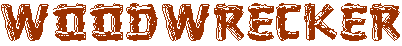# Useful Diagrams

Here are some useful diagrams related to woodworking efforts, etc.

Start by drawing the bottommost arc, which is a half-circle. Then draw the two diagonal lines from the points where the half-circle touches the horizontal line, both through the same point on the vertical line (the shape of the egg depends on the point you choose for this purpose). Draw the side arcs as shown, from the horizontal line to the diagonal lines. Draw the top arc between the two diagonal lines.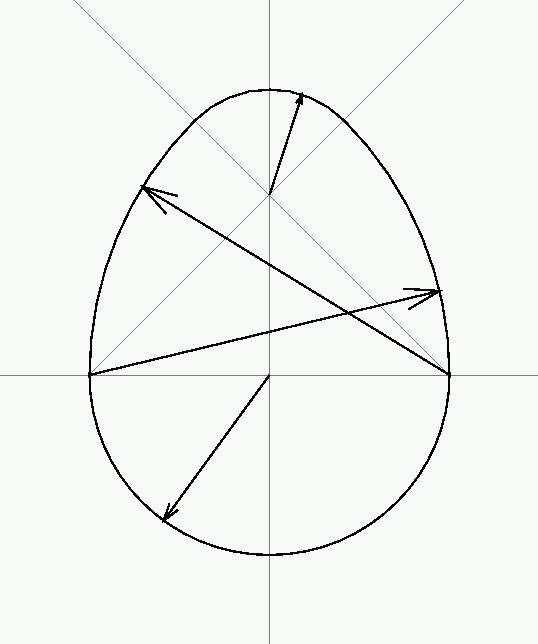Glue-up diagram for creating staves for a turned vessel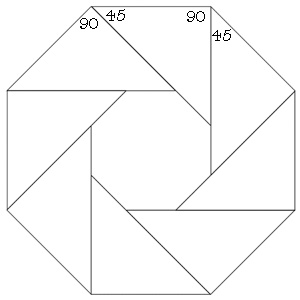Templates for 4, 5, 6, 7 and 8 sided shapes
Square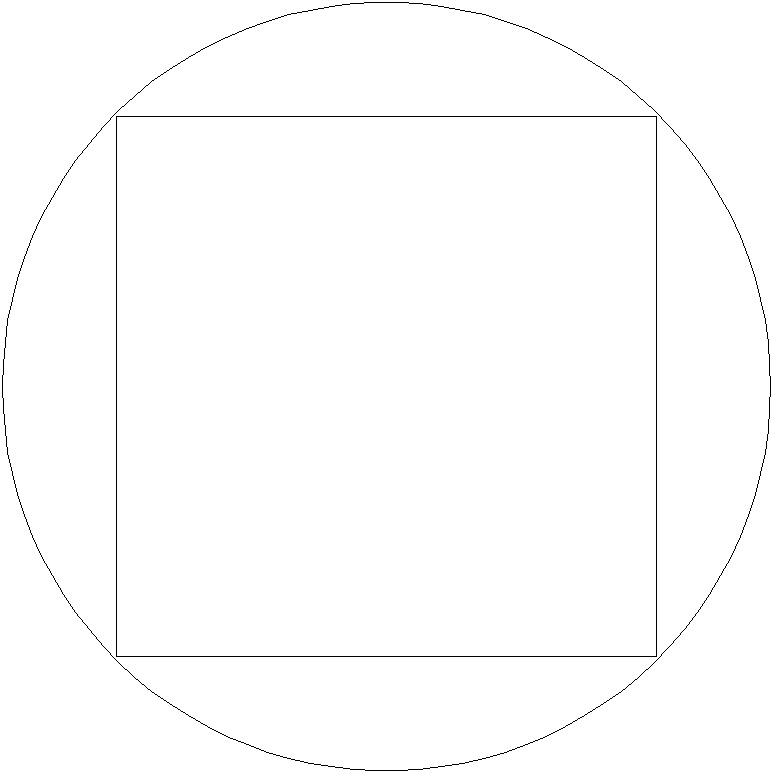Pentagon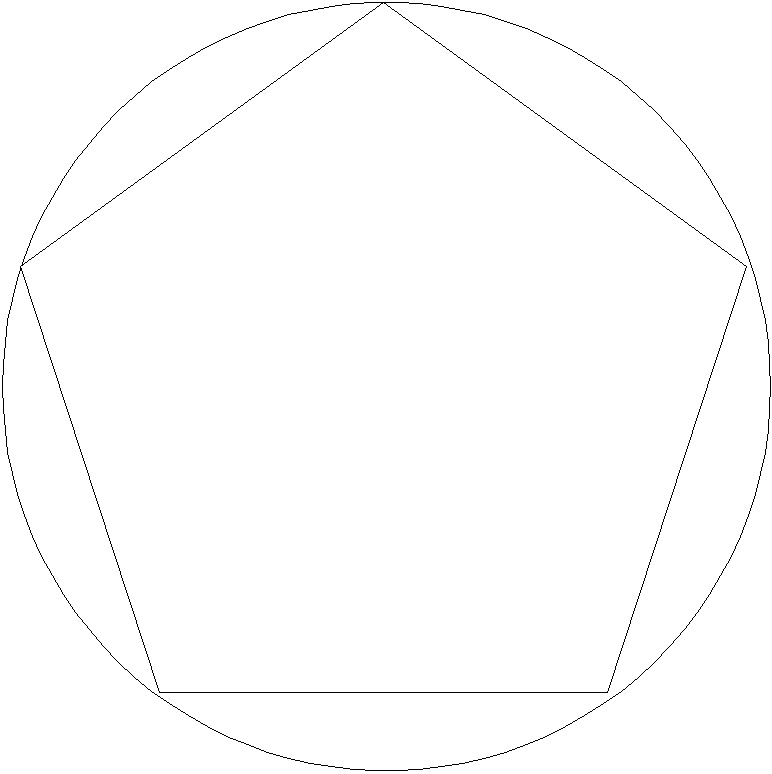Hexagon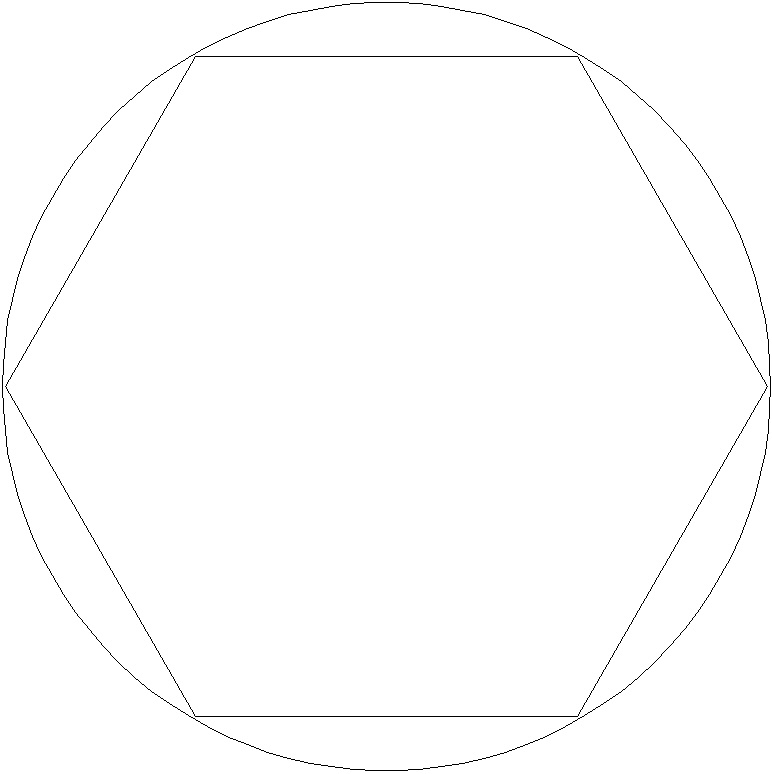Heptagon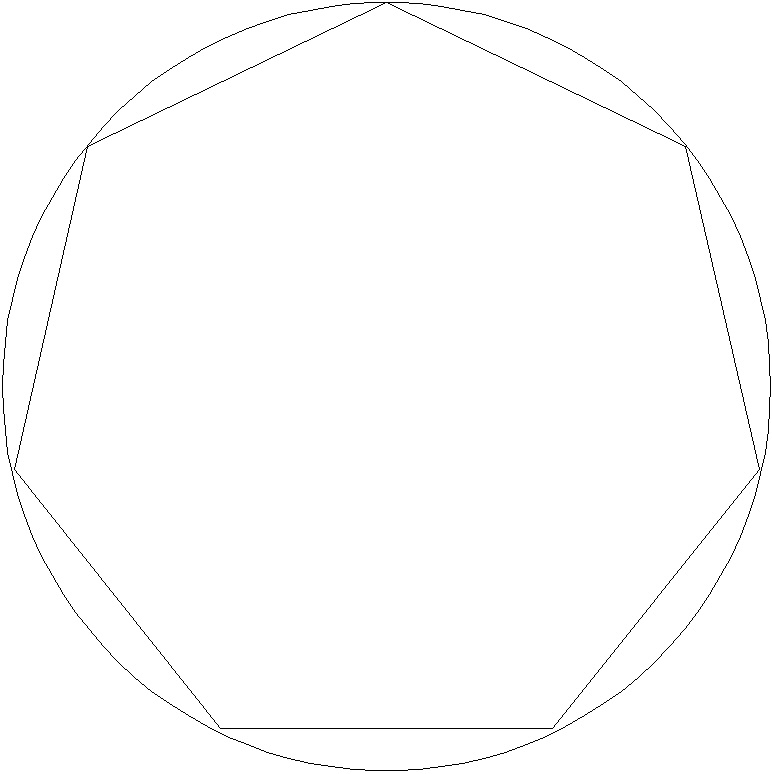Octagon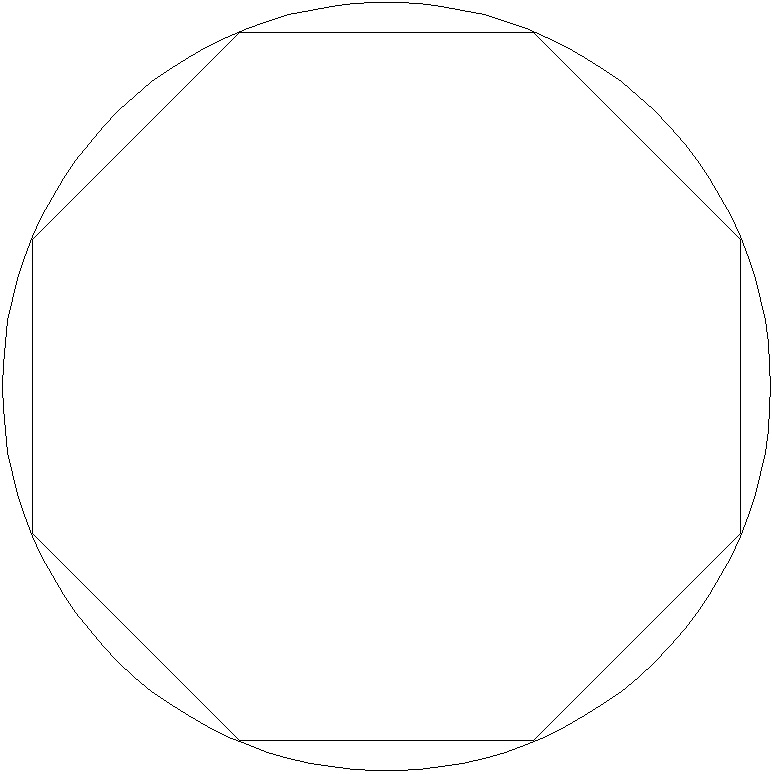Send mail to the WebMaster